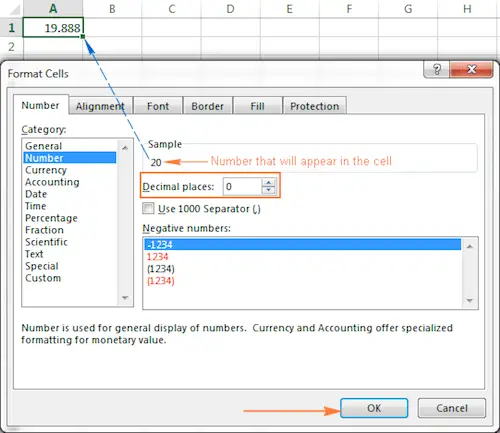# How To Round Numbers In Excel – ROUND, ROUNDUP, And ROUNDDOWN

On some occasions, when you don’t need an exact answer, rounding is a useful skill to use. In plain English, to round a number is to eliminate the least significant digits, making it simpler but keeping close to the original value. In other words, rounding lets you get an approximate number with the desired level of accuracy.

Discover the best calculators to help you round numbers.

In everyday life, rounding is commonly used to make numbers easier to estimate, communicate, or work with. For example, you can use rounding to make long decimal numbers shorter to report the results of complex calculations or round off currency values.

Many different ways of rounding exist, such as rounding to an integer, rounding to a specified increment, rounding to simple fractions, and so on.

Microsoft Excel provides a handful of functions to handle different rounding types.

## Excel Rounding By Changing The Cell Format

If you want to round numbers in Excel solely for presentations purposes, you can change the cell’s format by performing the following steps:

1. Select the cell with the number(s) you want to round.
2. Open the Format Cells dialog by pressing Ctrl + 1 or right click the cell(s) and choose Format Cells… from the context menu.
3. In the Format Cells window, switch to either Number or Currency tab, and type the number of decimal places you want to display in the Decimal paces box. A preview of the rounded number will immediately show up under Sample.
4. Click the OK button to save the changes and close the Format Cells dialog.

Learn more about the round half up and round half down modes.

## Excel Functions To Round Numbers

Unlike formatting options that change only the display value, Excel round functions alter the actual value in a cell.

## #1: Excel ROUND Function

ROUND is the major rounding function in Excel that rounds a numeric value to a specified number of digits.

Syntax: ROUND(number, num_digits)

Number – any real number you want to round. This can be a number, reference to a cell containing the number, or a formula-driven value.

Num_digits – the number of digits to round the number to. You can supply a positive or negative value in this argument:

• If num_digits is greater than 0, the number is rounded to the specified number of decimal places.
• For example =ROUND(15.55,1) rounds 15.55 to 15.6.
• If num_digits is less than 0, all decimal places are removed and the number is rounded to the left of the decimal point (to the nearest ten, hundred, thousand, etc.).
• For example =ROUND(15.55,-1) rounds 15.55 to the nearest 10 and returns 20 as the result.
• If num_digits equals 0, the number is rounded to the nearest integer (no decimal places).
• For example =ROUND(15.55,0) rounds 15.55 to 16.

The Excel ROUND function follows the general math rules for rounding, where the number to the right of the rounding digit determines whether the number is rounded upwards or downwards.

Rounding digit is the least significant digit retained once the number is rounded, and it gets changed depending on whether the digit that follows it is greater or less than 5:

• If the digit to the right of the rounding digit is 0, 1, 2, 3, or 4, the rounding digit is not changed, and the number is said to be rounded down.
• If the rounding digit is followed by 5, 6, 7, 8, or 9, the rounding digit is increased by one, and the number is rounded up.

The following screenshot demonstrates a few ROUND formula examples:

Discover more about the decimal place value.

## #2: Excel ROUNDUP Function:

The ROUNDUP function rounds the number upward (away from 0) to a specified number of digits.

Syntax: ROUNDUP(number, num_digits)

Number – the number to be rounded up.

Num_digits – the number of digits you want to round the number to. You can supply both positive and negative numbers in this argument, and it works like num_digits of the ROUND function discussed above, except that a number is always rounded upward.

What is the ROUND function?

## #3: Excel ROUNDDOWN Function:

The ROUNDDOWN function in Excel does the opposite of what ROUNDUP does, i.e. rounds a number down, toward zero.

Syntax: ROUNDDOWN(number, num_digits)

Number – the number to be rounded down.

Num_digits – the number of digits you want to round the number to. It works like the num_digits argument of the ROUND function, except that a number is always rounded downward.

Check out other useful calculators here

The following screenshot demonstrates the ROUNDDOWN function in action.

© 2022 rounding.to . Powered by WordPress. Theme by Viva Themes.